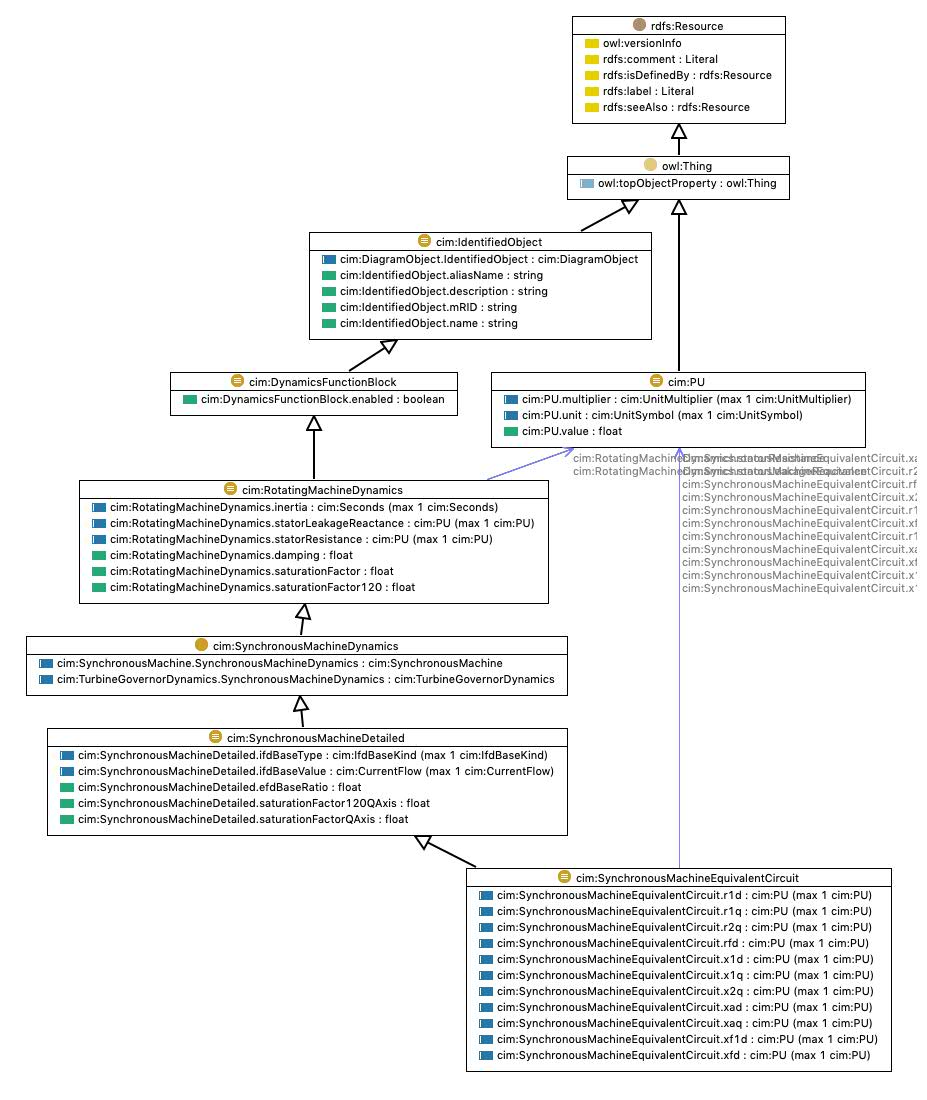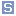## http://www.iec.ch/TC57/CIM#SynchronousMachineEquivalentCircuit Class cim:SynchronousMachineEquivalentCircuittypeClass [owl:Class]
commentThe electrical equations for all variations of the synchronous models are based on the SynchronousEquivalentCircuit diagram for the direct and quadrature axes. Equations for conversion between Equivalent Circuit and Time Constant Reactance forms: Xd = Xad + Xl Xd = Xl + Xad * Xfd / (Xad + Xfd) Xd = Xl + Xad * Xfd * X1d / (Xad * Xfd + Xad * X1d + Xfd * X1d) Xq = Xaq + Xl Xq = Xl + Xaq * X1q / (Xaq+ X1q) Xq = Xl + Xaq * X1q* X2q / (Xaq * X1q + Xaq * X2q + X1q * X2q) Tdo = (Xad + Xfd) / (omega0 * Rfd) Tdo = (Xad * Xfd + Xad * X1d + Xfd * X1d) / (omega0 * R1d * (Xad + Xfd) Tqo = (Xaq + X1q) / (omega0 * R1q) Tqo = (Xaq * X1q + Xaq * X2q + X1q * X2q)/ (omega0 * R2q * (Xaq + X1q) Same equations using CIM attributes from SynchronousMachineTimeConstantReactance class on left of = sign and SynchronousMachineEquivalentCircuit class on right (except as noted): xDirectSync = xad + RotatingMachineDynamics.statorLeakageReactance xDirectTrans = RotatingMachineDynamics.statorLeakageReactance + xad * xfd / (xad + xfd) xDirectSubtrans = RotatingMachineDynamics.statorLeakageReactance + xad * xfd * x1d / (xad * xfd + xad * x1d + xfd * x1d) xQuadSync = xaq + RotatingMachineDynamics.statorLeakageReactance xQuadTrans = RotatingMachineDynamics.statorLeakageReactance + xaq * x1q / (xaq+ x1q) xQuadSubtrans = RotatingMachineDynamics.statorLeakageReactance + xaq * x1q* x2q / (xaq * x1q + xaq * x2q + x1q * x2q) tpdo = (xad + xfd) / (2*pi*nominal frequency * rfd) tppdo = (xad * xfd + xad * x1d + xfd * x1d) / (2*pi*nominal frequency * r1d * (xad + xfd) tpqo = (xaq + x1q) / (2*pi*nominal frequency * r1q) tppqo = (xaq * x1q + xaq * x2q + x1q * x2q)/ (2*pi*nominal frequency * r2q * (xaq + x1q). Are only valid for a simplified model where "Canay" reactance is zero.
subClassOfcim:SynchronousMachineDetailed
equivalentClasscim:SynchronousMachineEquivalentCircuit.r1q max 1 cim:PUcim:SynchronousMachineEquivalentCircuit.rfd max 1 cim:PUcim:SynchronousMachineEquivalentCircuit.r1d max 1 cim:PUcim:SynchronousMachineEquivalentCircuit.xaq max 1 cim:PUcim:SynchronousMachineEquivalentCircuit.xfd max 1 cim:PUcim:SynchronousMachineEquivalentCircuit.xf1d max 1 cim:PUcim:SynchronousMachineEquivalentCircuit.xad max 1 cim:PUcim:SynchronousMachineEquivalentCircuit.r2q max 1 cim:PUcim:SynchronousMachineEquivalentCircuit.x1d max 1 cim:PUcim:SynchronousMachineEquivalentCircuit.x2q max 1 cim:PUcim:SynchronousMachineEquivalentCircuit.x1q max 1 cim:PU

Generated with TopBraid Composer by TopQuadrant, Inc.